Courses

# Test : Geometric Shapes - 1

## 10 Questions MCQ Test Mathematics for Class 5 (V) - CBSE and NCERT Curriculum | Test : Geometric Shapes - 1

Description
This mock test of Test : Geometric Shapes - 1 for Class 5 helps you for every Class 5 entrance exam. This contains 10 Multiple Choice Questions for Class 5 Test : Geometric Shapes - 1 (mcq) to study with solutions a complete question bank. The solved questions answers in this Test : Geometric Shapes - 1 quiz give you a good mix of easy questions and tough questions. Class 5 students definitely take this Test : Geometric Shapes - 1 exercise for a better result in the exam. You can find other Test : Geometric Shapes - 1 extra questions, long questions & short questions for Class 5 on EduRev as well by searching above.
QUESTION: 1

### A point is a mark of ______________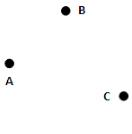Solution:

Point is a mark of position. A point specifies the exact location. Point is denoted by a dot (.)

QUESTION: 2

### A line contains ____________ number of points.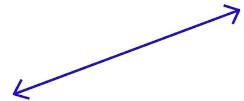Solution:

A line contains an infinite number of points.

QUESTION: 3

### The line AB is denoted by

Solution:

Line AB is denoted by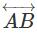since it extends infinitely on both sides.

QUESTION: 4

Two different lines in a plane having a common point are called ____________

Solution:

Intersecting lines are two lines that share exactly one point. This shared point is called the point of intersection.

QUESTION: 5

Three or more points in a plane are said to be collinear if they lie on the

Solution:

Three or more points that lie on the same line are collinear points.

QUESTION: 6

If A, B, C and D are collinear in the same sequence, then

Solution: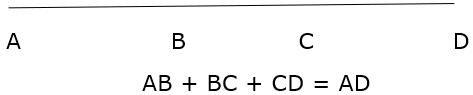QUESTION: 7

If AB=6cm,CD=4cm then AB+2CD is

Solution:

AB + 2CD = 6 + 2x4 = 14 cm

QUESTION: 8

If B is Mid-point of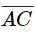then AC=______

Solution: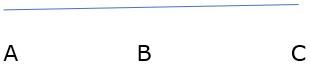As B is the mid-point of AC, AB = BC

AC = AB + BC

=> AC = 2AB

QUESTION: 9

A part of line with two end points is called a

Solution:

A part of line with two end points is called line segment.

QUESTION: 10

If AB = 6cm, CD = 3cm, the relation between AB and CD is ______

Solution:

Since 6cm > 3cm, therefore, AB>CD